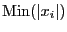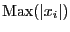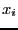Next: Kharitonov polynomials Up: Parametric polynomials and eigenvalues Previous: Largest square enclosed in   Contents

Condition number

The condition number of a polynomial may be defined either as the ratio lowest root over largest root or as the ratiooverwhereare the roots of the polynomial. In the later case the condition number has a value between 0 and 1. The minimal and maximal values of the condition number of a parametric polynomial in both form may be calculated using the procedure:

int ALIAS_Min_Max_CN(int Degree,
int Nb_Parameter,INTERVAL_VECTOR (* TheCoeff)(INTERVAL_VECTOR &),
int Nb_Constraints,INTEGER_VECTOR &Type_Eq,
int (* TheMatrix)(INTERVAL_VECTOR &, INTERVAL_MATRIX &),
int Has_Matrix,
INTERVAL_VECTOR (* IntervalFunction)(int,int,INTERVAL_VECTOR &),
INTERVAL & TheDomain,INTERVAL_VECTOR & TheDomain_Parameter, int Type,
int Nb_Points,int Absolute,
int rand,int Iteration,
double Accuracy_Variable,double Accuracy,double AccuracyM,
INTERVAL &Lowest,INTERVAL &Highest,
INTERVAL_MATRIX &Place,int  Stop, double *Seuil,
int (* Solve_Poly)(double *, int *,double *),
int (* Simp_Proc)(INTERVAL_VECTOR &))
where the arguments are identical than for the previous procedure except for:
• Absolute: 0 if looking for the ratio minimal root over maximal root, 1 if looking for the ratio in absolute value
• AccuracyM: the accuracy with which the ratio will be computed
• Lowest, Highest: minimal and maximal value of the condition number
This procedure allows for the calculation of the minimal and maximal condition number of a matrix. Various bisection methods are available through the use of the integer Single_Bisection:
• 1: mode 1 of section 2.4.1.3
• 2: mode 6 of section 2.4.1.3 if the gradient is not available
• 3,4: mode 1 of section 2.4.1.3
• 5: mode 5 of section 2.4.1.3
A specific procedure is used when the gradient is available. If the interval for the root includes 0 we bisect it. Otherwise we use the smear function to decide which other variable should be bisected.Next: Kharitonov polynomials Up: Parametric polynomials and eigenvalues Previous: Largest square enclosed in   Contents
Jean-Pierre Merlet 2012-12-20